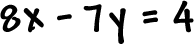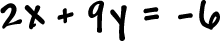We'd start Cramer's Rule by finding D:and we'd get stuck withand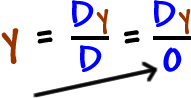BIG TROUBLE!

So, this all ties together!

If the determinant of the coefficient matrix is zero, then the system does not have a nice ( x , y ) solution.  (Remember that the lines could be parallel, or they could be the same line.)  AND, if the determinant of a matrix is zero, then the matrix does not have an inverse...  So, we can't get a nice ( x , y ) solution!

(and be sure to do a quick check on the determinant before you start in on the inverse):

Use inverse matrices to solve: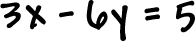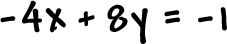Use inverse matrices to solve: